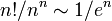# Full transformation monoid

This is a variation of symmetric group|Find other variations of symmetric group |

## Definition

### For a set

The full transformation monoid or full transformation semigroup on a set$S$ is the monoid defined as follows:

1. The elements of the monoid are functions from$S$ to itself.
2. The identity element of the monoid is the identity map from$S$ to itself.
3. The monoid operation (multiplication) is composition of functions.

### For a degree

The full transformation monoid of degree$n$ is defined as the full transformation monoid on a set of size$n$. Any bijection between sets induces an isomorphism between their full transformation monoids, so the full transformation monoid of degree$n$ is uniquely defined up to isomorphism.

## Facts

### Order

For finite$n$, the full transformation monoid of degree$n$ has order$n^n$.

### Group of invertible elements

The group of invertible elements in the full transformation monoid is precisely the symmetric group on the underlying set, i.e., the group of bijective maps from the underlying set to itself. For finite$n$, the size of this group is$n! = n \cdot (n-1) \cdot \dots \cdot 2 \cdot 1$. As a fraction, we have that the fraction of invertible elements in the full transformation monoid is$\! n!/n^n \sim 1/e^n$, which goes to infinity as$n \to \infty$.